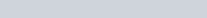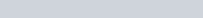# Galilean invariance of the wave equation

This post follows James Rohlf’s Modern Physics from α to Z0 (p.104-105). See also the slides here.

The Galilean transformations are applied here to 3D space and 3D time in this case because both space and time are independent arguments. Start with the standard configuration for relativity in which motion is parallel to the x-t axis. The space Galilean transformation isThe dual time Galilean transformation iswhere r, s, and t are time axes and w = 1/v. The y, z, s, and r axes are trivially invariant. Consider the one-dimensional wave equation for an electric field in a vacuum:

The first space derivative of the Galilean transformation is as follows:

The second space derivative of the Galilean transformation is as follows:

The first time derivative of the Galilean transformation is as follows:

The second time derivative of the Galilean transformation is as follows:

The full result is as follows:

The conclusion is that the one-dimensional wave equation for an electric field in a vacuum is form invariant under the Galilean transformations with space and time duality.